# GMAT Math : Calculating whether acute / obtuse triangles are congruent

## Example Questions

### Example Question #7 : Acute / Obtuse Triangles

Is it true that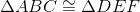?

Suppose you want to answer this question, and you know that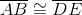and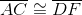. Which of the following additional facts would help you to answer this question one way or the other?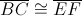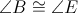None of these statements would be sufficient to answer the question.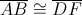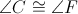Explanation:

If you know either that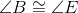or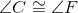, you have three congruencies - two sides and a nonincluded angle. This is not enough to establish triangle congruence,

If you know that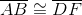, this, along with the other two statements, establishes that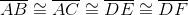; all this proves is that both triangles are isosceles.

If you also know that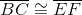, however, the three statements together make three side congruencies, setting up the Side-Side-Side criterion for triangle congruence.

### Example Question #8 : Acute / Obtuse Triangles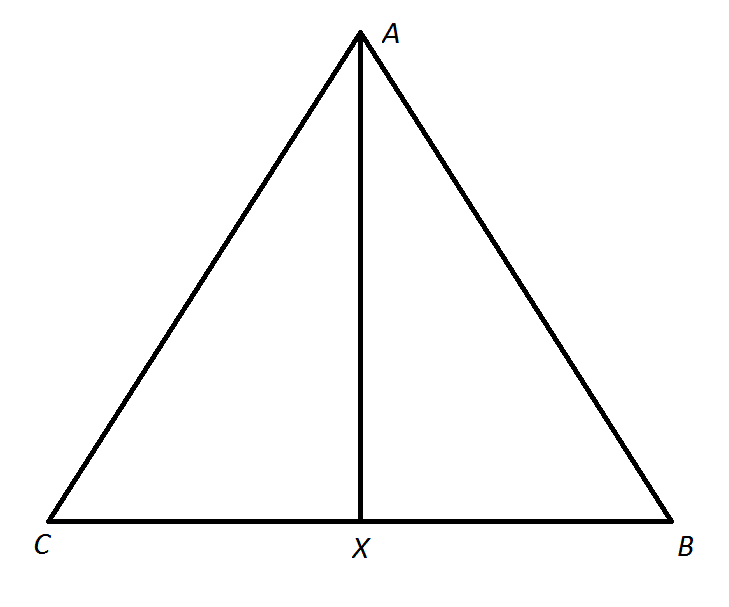Note: Figure NOT drawn to scale

Refer to the above diagram. Which of the following statements is NOT a consequence of the fact that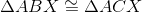?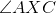is a right angleis the midpoint of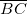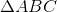is an equilateral triangle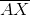bisects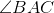is an altitude ofis an equilateral triangle

Explanation:

We use the congruence of corresponding sides and angles of congruent triangles to prove four of these statements: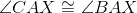, sobisects.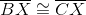, sois the midpoint of.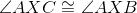, and, since they form a linear pair, they are supplementary - therefore, they are right angles. Also, by definition, this makesan altitude.

However, nothing in this congruence proves thatis congruent to the other two sides of(which are congruent). The correct statement to exclude is thatis equilateral.

Tired of practice problems?

Try live online GMAT prep today.